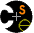CSE 401,  Sp '04:  PL/0 - Original Syntax Description

The following grammar describes the base version of the PL/0 language, i.e., PL/0 as implemented by the base compiler distributed to you at the begining of the quarter.

It uses some syntactic sugar that is not strictly BNF, e.g. braces {} mean "0 or more", and brackets [ ] mean "optional".

You might also want to visit the extended BNF (but this link won't work until after HW#2 is turned in).

 Program ::= module Id ; Block Id . Block ::= DeclList begin StmtList end DeclList ::= { Decl ; } Decl ::= ConstDecl | VarDecl | ProcDecl ConstDecl ::= const ConstDeclItem { , ConstDeclItem } ConstDeclItem ::= Id : Type = ConstExpr ConstExpr ::= Id | Integer VarDecl ::= var VarDeclItem { , VarDeclItem } VarDeclItem ::= Id : Type ProcDecl ::= procedure Id ( [ FormalDecl {, FormalDecl }] ) ; Block Id FormalDecl ::= Id : Type Type ::= int StmtList ::= { Stmt ; } Stmt ::= CallStmt | AssignStmt | OutStmt | IfStmt | WhileStmt CallStmt ::= Id ( [ Exprs ] ) AssignStmt ::= LValue := Expr LValue ::= Id OutStmt ::= output := Expr IfStmt ::= if Test then StmtList end WhileStmt ::= while Test do StmtList end Test ::= odd Sum | Sum Relop Sum Relop ::= < | <= | = | >= | > | <> Exprs ::= Expr { , Expr } Expr ::= Sum Sum ::= Term { (+ | -) Term } Term ::= Factor { (* | /) Factor } Factor ::= - Factor | LValue | Integer | input | ( Expr )Computer Science & Engineering University of Washington Box 352350 Seattle, WA  98195-2350 (206) 543-1695 voice, (206) 543-2969 FAX [comments to cse401-webmaster at cs.washington.edu]